# How to gracefully moving objects - Unity 】 【 8 method

2022-09-23 08:50:29

Unity The engine also provides numerous methods,The usage habits of each developer are also different,So it's often not clear which approach is best or most effective in this scenario.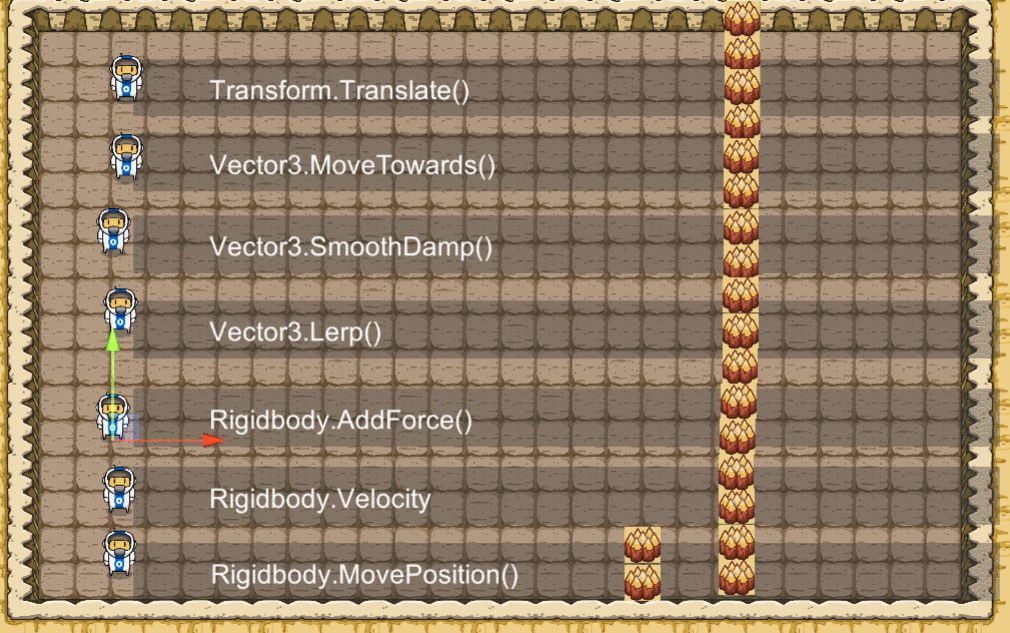## 项目地址

How to move objects gracefully？8个方法

## move in a certain direction

### Transform.Position

``````transform.position = new Vector3(2, 1, 0);
``````

When we assign a new coordinate to the object every frame,那么看起来,This object is in motion.

``````    void Update()
{

var dir = new Vector3(0.02f, 0, 0);
transform.position += dir;
}
``````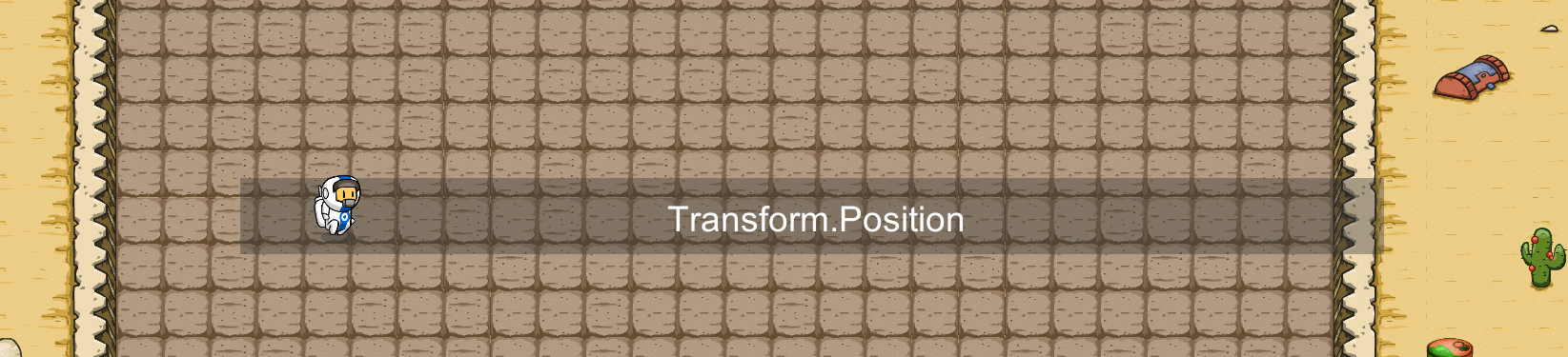### Transform.Translate()

due to direct change Position Properties don't look very elegant.所以 `Transform` Components provide a friendlier approach：`Transform.Translate()` .

``````      void Update()
{

var dir = new Vector3(0.02f, 0, 0);
transform.Translate(dir);
}
``````

`````` public void Translate(Vector3 translation, [DefaultValue("Space.Self")] Space relativeTo)
{

if (relativeTo == Space.World)
this.position += translation;
else
this.position += this.TransformDirection(translation);
}
``````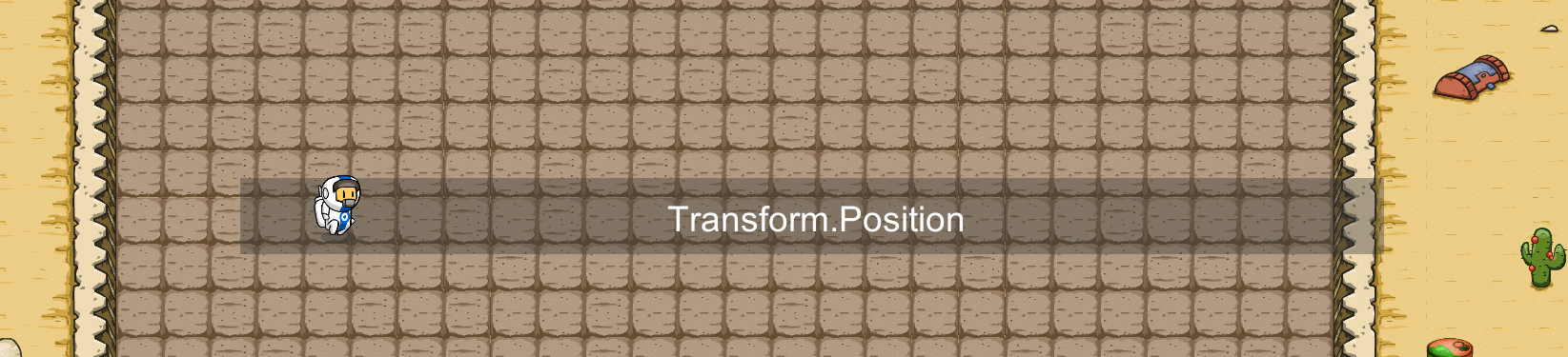But this approach created a problem.Due to differences between devices or changes in dynamic data, the interval between each frame will be unequal,因此,If the object movement is controlled by the number of frames,There is no way to accurately grasp the moving distance of an object.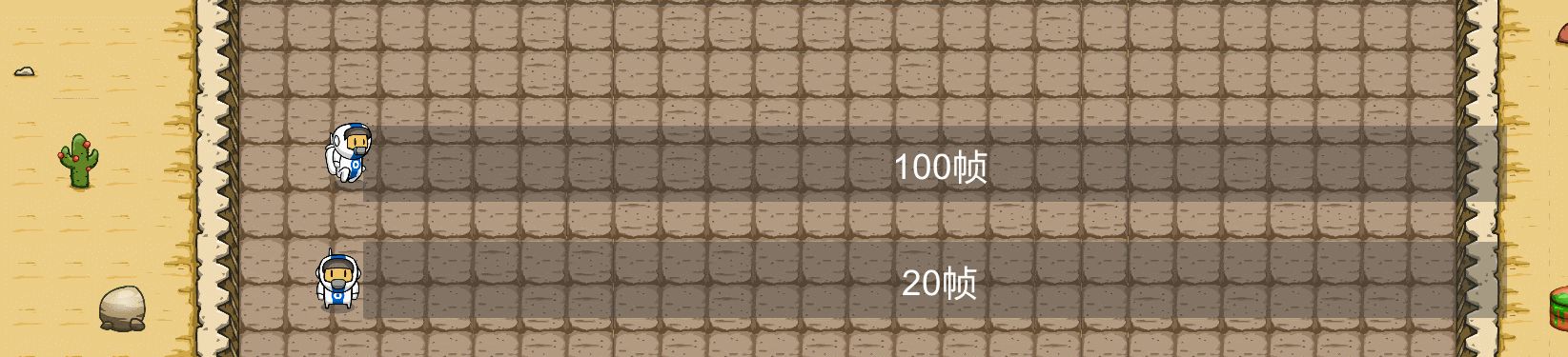So we need to multiply by the original `Time.deltaTime`属性的值,This ensures that the distance moved per second is consistent.

``````void Update()
{

var dir = new Vector3(2f, 0, 0)*time;
transform.Translate(dir);
}
``````

In this way, the moving distance of different frame numbers will be the same.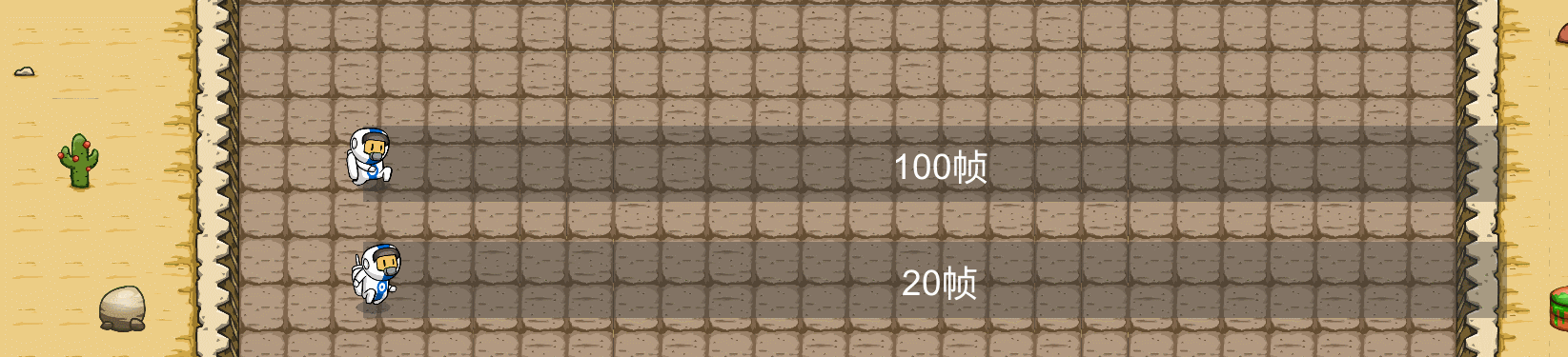But that's not elegant enough.在游戏中,We often need to change the speed of an object.为了方便实现,We usually use the unit vector to determine the direction,Add a float value to control the speed.

``````public float speed = 2;
void Update()
{

var dir = new Vector3(2,0,0)
transform.Translate(dir.normalized * speed * Time.deltaTime);
}
``````

## 移动到指定位置

1. 速度：The object moves towards the target with a certain speed.
2. 时间：The object reaches the target in time.

### Vector3.MoveTowards()：固定速度

Move to the target position at a fixed speed

``````public Vector3 targetPosition;
public float speed=10;
void Update()
{

transform.position = Vector3.MoveTowards(transform.position, targetPosition, speed * Time.deltaTime);
}
``````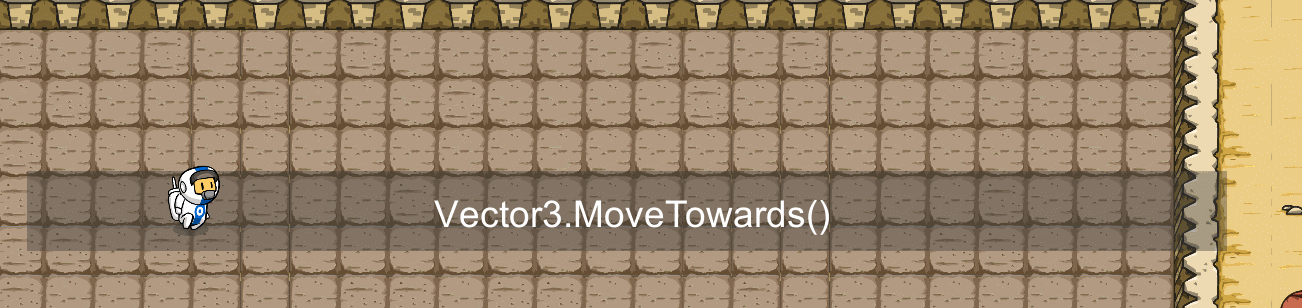### Vector3.SmoothDamp()：平滑移动

``````public Vector3 targetPosition;
public float smoothTime = 0.5f;
public float speed = 10;
Vector3 velocity ;
void Update()
{

transform.position = Vector3.SmoothDamp(transform.position, targetPosition, ref velocity, smoothTime, speed);
}
``````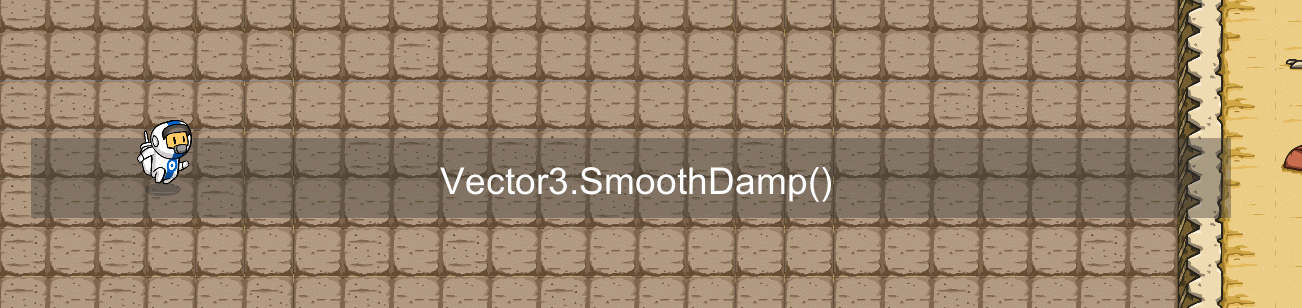### Vector3.Lerp()：Linear time movement

The method is meant to be during the invocation of the method,Elapsed time divided by total duration,Get the current displacement target.

`````` // 终点
public Vector3 targetPosition;
// 开始位置
public Vector3 startPosition;
// 持续时间
public float lerpDuration = 4;
// 记录运行时间
private float _timeElapsed = 0;

void Start()
{

startPosition = transform.position;
}

void Update()
{

// 记录下一个位置
Vector3 valueToLerp;
_timeElapsed += Time.deltaTime;
if (_timeElapsed < lerpDuration)
{

valueToLerp  = Vector3.Lerp(startPosition, targetPosition, _timeElapsed / lerpDuration);
}
else
{

valueToLerp = targetPosition;
}
transform.position = valueToLerp;
}
``````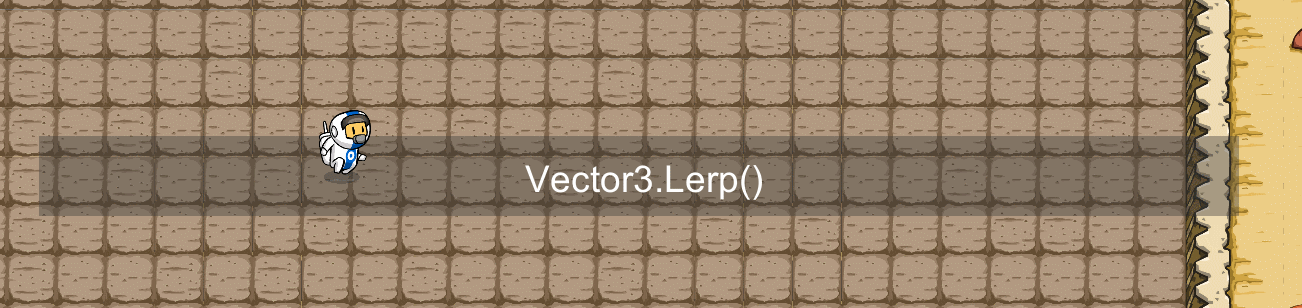The above methods are enough to allow us to accurately and freely manipulate the movement of objects.
But there are some scenarios,我们并Such precise or consistent motion trajectories are not desired,We want the movement of objects to be affected Unity The physics engine influence or other object influence.
At the same time, if you use the above method to move,在 Unity Jitter occurs under the physics engine,Strange phenomena like going through rigid bodies.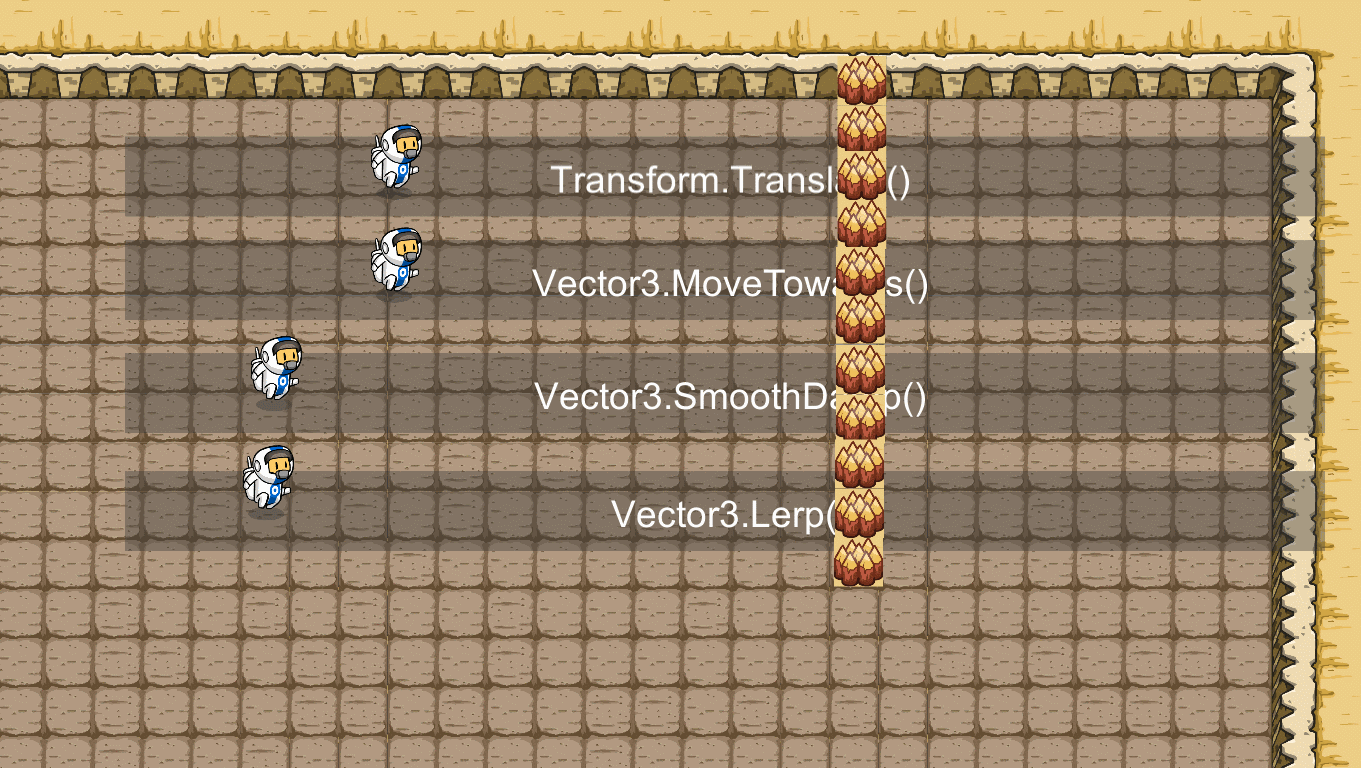## Physics engine moves

Use this method to add a directional force to an object.在力的作用下,The object will move.Then the movement speed and displacement will be related to the physical effects,such as the mass of an object,阻力,There's even gravity.

Gives a force to the object initially,Let it move according to the laws of physics.Use the scene to jump or collide normally.

``````    // 赋予200的力
public float force = 200;
private Rigidbody2D _rigidbody2D;
// 移动方向
private Vector3 dir = Vector3.right;

void Start()
{

_rigidbody2D = GetComponent<Rigidbody2D>();
}

``````

In order to better demonstrate the motion of the rigid body,I also gave the Rigidbody Linear Resistance instead1,In this way, there is no continuous external force applied,Objects stop because of friction.The object can be seen to stop very quickly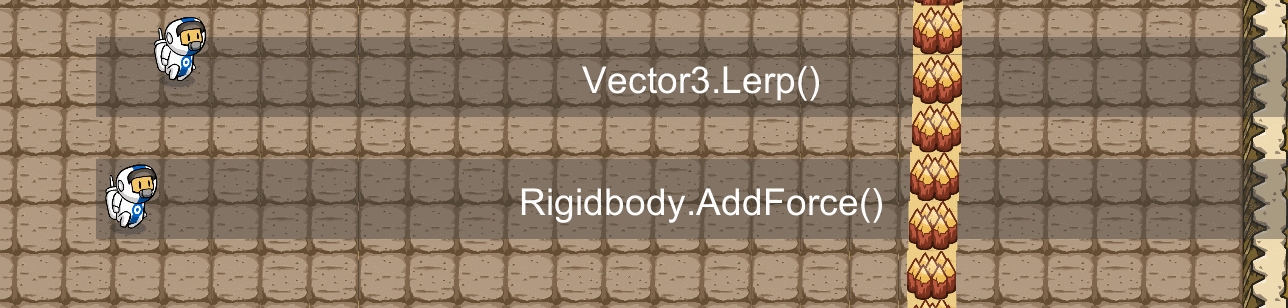`````` // 赋予2的力
public float force = 2;
private Rigidbody2D _rigidbody2D;
// 移动方向
private Vector3 dir = Vector3.right;
void Start()
{

_rigidbody2D = GetComponent<Rigidbody2D>();
}

void FixedUpdate()
{

}
``````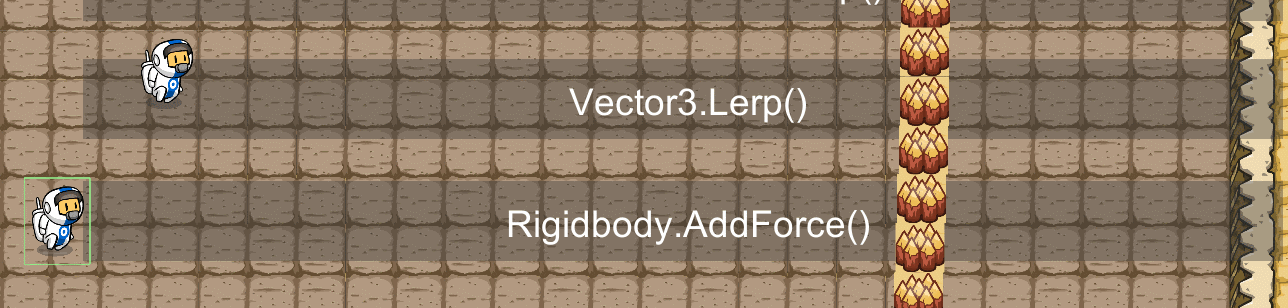You can see from the effect,under continuous external force,Objects move faster and faster,But it will stop before the block.

### Rigidbody.Velocity

``````    public float speed = 10;
private Rigidbody2D _rigidbody2D;
// 移动方向
private Vector3 dir = Vector3.right;

void Start()
{

_rigidbody2D = GetComponent<Rigidbody2D>();
}

void FixedUpdate()
{

_rigidbody2D.velocity = dir * speed;
}
``````

Seeing that objects already have speed from the start,而通过`AddForce`method to add a force to the object,The speed is gradually increased.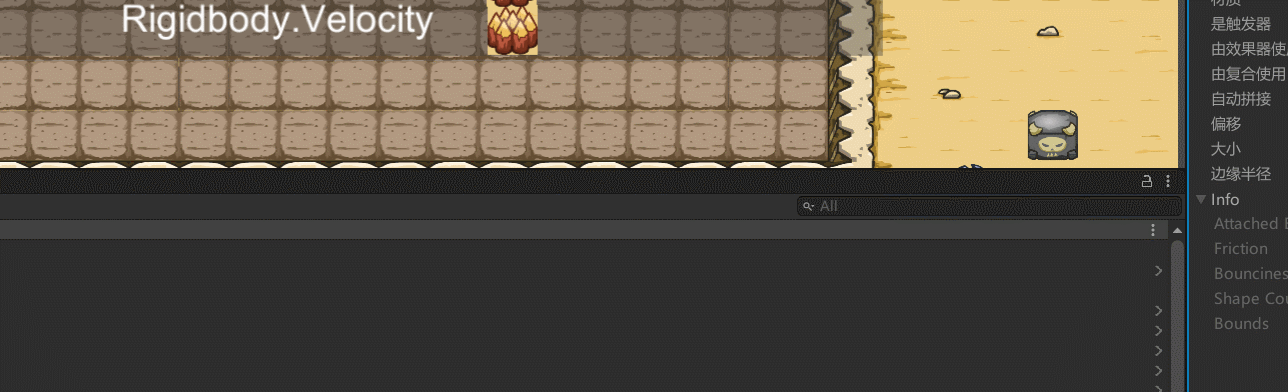### Rigidbody.MovePosition()

This method has relatively limited usage scenarios,When the rigid body type of the object is Kinematic 时,使用`Rigidbody.MovePosition()` 方法进行移动.

``````	public float speed = 10;
private Rigidbody2D _rigidbody2D;
// 移动方向
private Vector3 dir = Vector3.right;

void Start()
{

_rigidbody2D = GetComponent<Rigidbody2D>();
}

private void FixedUpdate()
{

var positon = dir * (speed * Time.deltaTime);
_rigidbody2D.MovePosition(transform.position + positon);
}
``````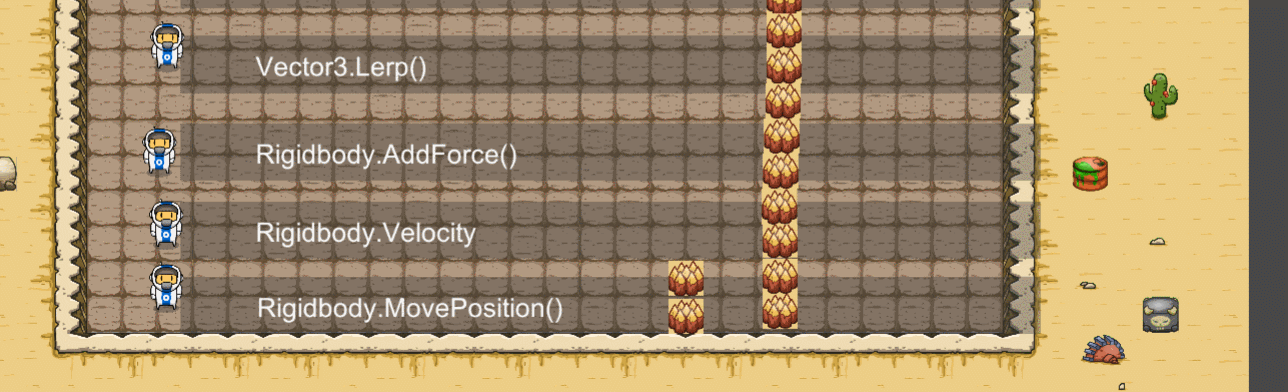https://chowdera.com/2022/266/202209230836030591.html